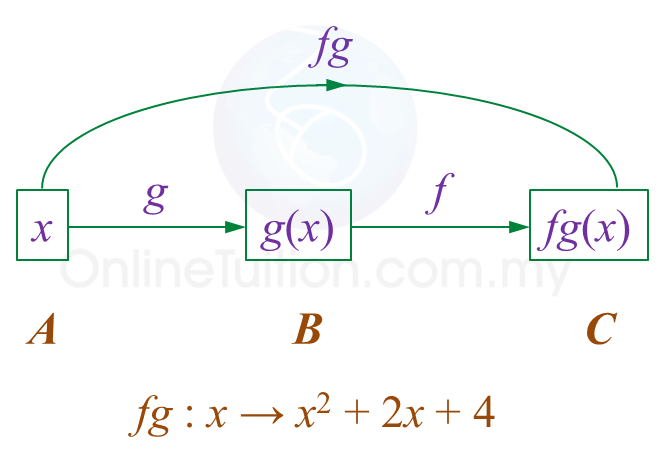# SPM Additional Mathematics 2018, Paper 1 (Question 20 – 22)

Question 20 (3 marks):
It is given that the curve y = (p – 2)x2x + 7, where p is a constant, intersects with the straight line y = 3x + 5 at two points.
Find the range of values of p.

Solution:

Question 21 (3 marks):
It is given that the quadratic equation hx2 – 3x + k = 0, where h and k are constants has roots β and 2β.
Express h in terms of k.

Solution:

Question 22 (4 marks):
Diagram 9 shows the relation between set A, set B and set C.Diagram 9

It is given that set A maps to set B by the function $\frac{x+1}{2}$ and maps to set C by fg : xx2 + 2x + 4.
(a) Write the function which maps set A to set B by using the function notation.
(b) Find the function which maps set B to set C.

Solution:(a)
$g:x\to \frac{x+1}{2}$

(b)